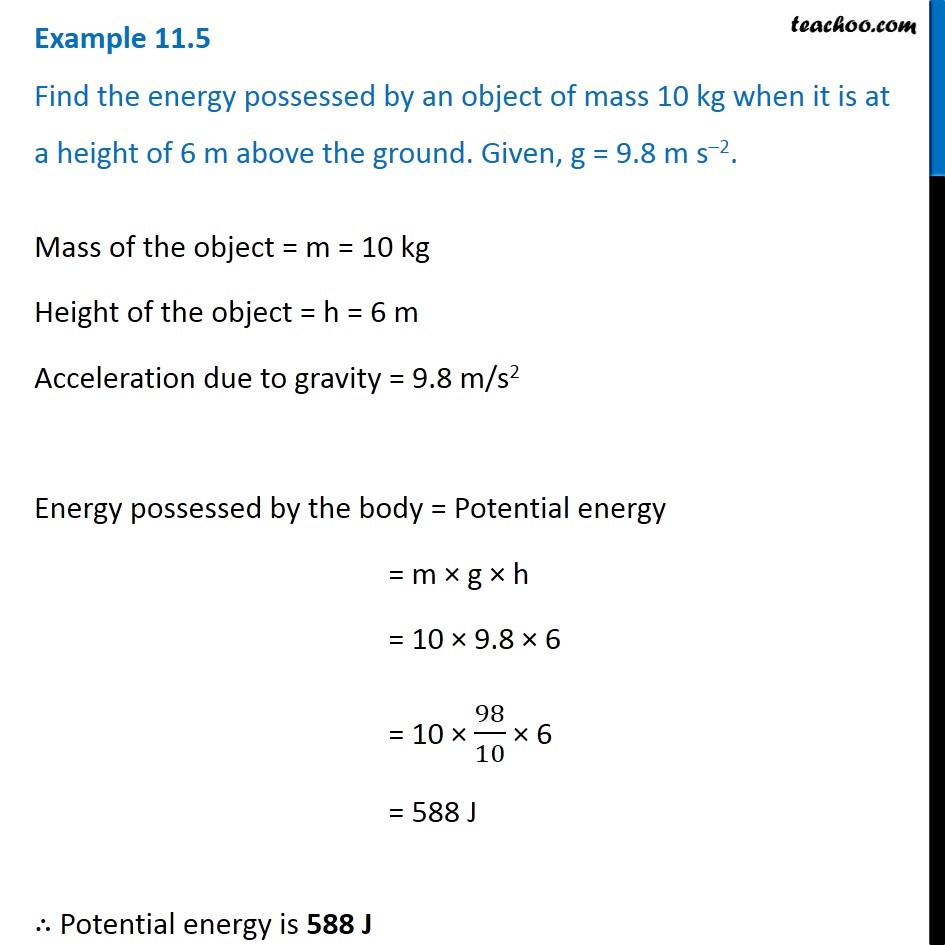Examples from NCERT Book

Class 9
Chapter 11 Class 9 - Work and EnergyLearn in your speed, with individual attention - Teachoo Maths 1-on-1 Class

### Transcript

Example 11.5 Find the energy possessed by an object of mass 10 kg when it is at a height of 6 m above the ground. Given, g = 9.8 m s–2. Mass of the object = m = 10 kg Height of the object = h = 6 m Acceleration due to gravity = 9.8 m/s2 Energy possessed by the body = Potential energy = m × g × h = 10 × 9.8 × 6 = 10 × 98/10 × 6 = 588 J ∴ Potential energy is 588 J